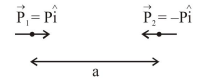# Two identical electric point dipoles have dipole moments

Question:

Two identical electric point dipoles have dipole moments $\vec{p}_{1}=p \hat{i}$ and $\vec{p}_{2}=-p \hat{i}$ and are held on the $x$ axis at distance 'a' from each other. When released, they move along the $x$-axis with the direction of their dipole moments remaining unchanged. If the mass of each dipole is'm', their speed when they arc infinitely far apart is:

1. $\frac{p}{a} \sqrt{\frac{1}{\pi \varepsilon_{0} m a}}$

2. $\frac{\mathrm{p}}{\mathrm{a}} \sqrt{\frac{3}{2 \pi \varepsilon_{0} \mathrm{ma}}}$

3. $\frac{\mathrm{p}}{\mathrm{a}} \sqrt{\frac{1}{2 \pi \varepsilon_{0} \mathrm{ma}}}$

4. $\frac{\mathrm{p}}{\mathrm{a}} \sqrt{\frac{2}{\pi \varepsilon_{0} \mathrm{ma}}}$

Correct Option: , 3

Solution:

Using energy conservation:

$\mathrm{KE}_{\mathrm{i}}+\mathrm{PE}_{\mathrm{i}}=\mathrm{KE}_{\mathrm{f}}+\mathrm{PE}_{\mathrm{f}}$$\mathrm{O}+\frac{2 \mathrm{KP}}{\mathrm{a}^{3}} \times \mathrm{P}=\frac{1}{2} \mathrm{mv}^{2} \times 2+0$

$V=\sqrt{\frac{2 P^{2}}{4 \pi \varepsilon_{0} a^{3} m}}=\frac{P}{a} \sqrt{\frac{1}{2 \pi \varepsilon_{0} a m}}$